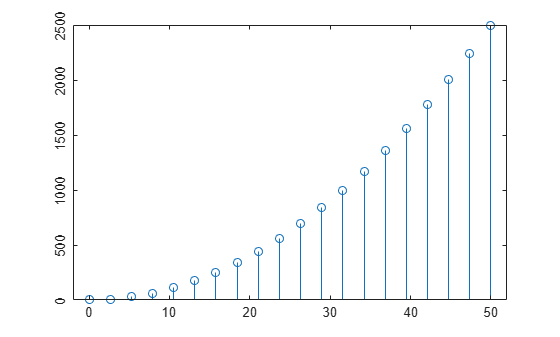# ytickangle

Rotate y-axis tick labels

## Syntax

``ytickangle(angle)``
``ytickangle(ax,angle)``
``ang = ytickangle``
``ang = ytickangle(ax)``

## Description

example

````ytickangle(angle)` rotates the y-axis tick labels for the current axes to the specified angle in degrees, where `0` is horizontal. Specify a positive value for counterclockwise rotation or a negative value for clockwise rotation.```

example

````ytickangle(ax,angle)` rotates the tick labels for the axes specified by `ax` instead of the current axes.```

example

````ang = ytickangle` returns the rotation angle for the y-axis tick labels of the current axes as a scalar value in degrees. Positive values indicate counterclockwise rotation. Negative values indicate clockwise rotation.```
````ang = ytickangle(ax)` uses the axes specified by `ax` instead of the current axes.```

## Examples

collapse all

Create a stem chart and rotate the y-axis tick labels 90 degrees so that they appear vertically.

```x = linspace(0,50,20); y = x.^2; stem(x,y) ytickangle(90)```Starting in R2019b, you can display a tiling of plots using the `tiledlayout` and `nexttile` functions. Call the `tiledlayout` function to create a 2-by-1 tiled chart layout. Call the `nexttile` function to create the axes objects `ax1` and `ax2`. Plot into each of the axes. Then rotate the y-axis tick labels for the lower plot by specifying `ax2` as the first input argument to `ytickangle`.

```tiledlayout(2,1) ax1 = nexttile; plot(ax1,rand(6)) ax2 = nexttile; plot(ax2,rand(6)) ytickangle(ax2,45)```Create a stem chart. Then, query the rotation angle for the y-axis tick labels. By default, the labels are not rotated.

```x = linspace(0,50,20); y = x.^2; stem(x,y)````ang = ytickangle`
```ang = 0 ```

## Input Arguments

collapse all

Rotation of tick labels, specified as a scalar value in degrees, where 0 is horizontal.

Example: `ytickangle(90)`

Target axes, specified as an `Axes` object or an array of `Axes` objects.

If you do not specify this argument, then `ytickangle` modifies the current axes.

## Algorithms

The `ytickangle` function sets and queries the `YTickLabelRotation` property of the `Axes` object.

## Version History

Introduced in R2016b